In this tutorial, we will discuss the concept of weight initialization, or more simply, how we initialize our weight matrices and bias vectors.

This tutorial is not meant to be a comprehensive initialization technique; however, it does highlight popular methods, but from neural network literature and general rules-of-thumb. To illustrate how these weight initialization methods work I have included basic Python/NumPy-like pseudocode when appropriate.#### Looking for the source code to this post?

Jump Right To The Downloads Section

### Constant Initialization

When applying constant initialization, all weights in the neural network are initialized with a constant value, C. Typically C will equal zero or one.

To visualize this in pseudocode let’s consider an arbitrary layer of a neural network that has 64 inputs and 32 outputs (excluding any biases for notional convenience). To initialize these weights via NumPy and zero initialization (the default used by Caffe, a popular deep learning framework) we would execute:

`>>> W = np.zeros((64, 32))`

Similarly, one initialization can be accomplished via:

`>>> W = np.ones((64, 32))`

We can apply constant initialization using an arbitrary of C using:

`>>> W = np.ones((64, 32)) * C`

Although constant initialization is easy to grasp and understand, the problem with using this method is that it’s near impossible for us to break the symmetry of activations (Heinrich, 2015). Therefore, it is rarely used as a neural network weight initializer.

### Uniform and Normal Distributions

A uniform distribution draws a random value from the range `[lower, upper]` where every value inside this range has equal probability of being drawn.

Again, let’s presume that for a given layer in a neural network we have 64 inputs and 32 outputs. We then wish to initialize our weights in the range `lower=-0.05` and `upper=0.05`. Applying the following Python + NumPy code will allow us to achieve the desired normalization:

`>>> W = np.random.uniform(low=-0.05, high=0.05, size=(64, 32))`

Executing the code above NumPy will randomly generate 64×32 = 2,048 values from the range [−0.05, 0.05], where each value in this range has equal probability.

We then have a normal distribution where we define the probability density for the Gaussian distribution as:

(1)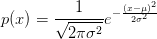$p(x) = displaystylefrac{1}{displaystylesqrt{2pisigma^{2}}}e^{-frac{(x - mu)^{2}}{2sigma^{2}}}$

The most important parameters here are µ (the mean) and σ (the standard deviation). The square of the standard deviation, σ2, is called the variance.

When using the Keras library the `RandomNormal` class draws random values from a normal distribution with µ = 0 and σ = 0.05. We can mimic this behavior using NumPy below:

`>>> W = np.random.normal(0.0, 0.05, size=(64, 32))`

Both uniform and normal distributions can be used to initialize the weights in neural networks; however, we normally impose various heuristics to create “better” initialization schemes (as we’ll discuss in the remaining sections).

### LeCun Uniform and Normal

If you have ever used the Torch7 or PyTorch frameworks you may notice that the default weight initialization method is called “Efficient Backprop,” which is derived by the work of LeCun et al. (1998).

Here, the authors define a parameter Fin (called “fan in,” or the number of inputs to the layer) along with Fout (the “fan out,” or number of outputs from the layer). Using these values we can apply uniform initialization by:

```>>> F_in = 64
>>> F_out = 32
>>> limit = np.sqrt(3 / float(F_in))
>>> W = np.random.uniform(low=-limit, high=limit, size=(F_in, F_out))```

We can also use a normal distribution as well. The Keras library uses a truncated normal distribution when constructing the lower and upper limits, along with a zero mean:

```>>> F_in = 64
>>> F_out = 32
>>> limit = np.sqrt(1 / float(F_in))
>>> W = np.random.normal(0.0, limit, size=(F_in, F_out))```

### Glorot/Xavier Uniform and Normal

The default weight initialization method used in the Keras library is called “Glorot initialization” or “Xavier initialization” named after Xavier Glorot, the first author of the paper, Understanding the difficulty of training deep feedforward neural networks.

For the normal distribution the `limit` value is constructed by averaging the Fin and Fout together and then taking the square-root (Jones, 2016). A zero-center (µ = 0) is then used:

```>>> F_in = 64
>>> F_out = 32
>>> limit = np.sqrt(2 / float(F_in + F_out))
>>> W = np.random.normal(0.0, limit, size=(F_in, F_out))```

Glorot/Xavier initialization can also be done with a uniform distribution where we place stronger restrictions on `limit`:

```>>> F_in = 64
>>> F_out = 32
>>> limit = np.sqrt(6 / float(F_in + F_out))
>>> W = np.random.uniform(low=-limit, high=limit, size=(F_in, F_out))```

Learning tends to be quite efficient using this initialization method and I recommend it for most neural networks.

### He et al./Kaiming/MSRA Uniform and Normal

Often referred to as “He et al. initialization,” “Kaiming initialization,” or simply “MSRA initialization,” this technique is named after Kaiming He, the first author of the paper, Delving Deep into Rectifiers: Surpassing Human-Level Performance on ImageNet Classification.

We typically use this method when we are training very deep neural networks that use a ReLU-like activation function (in particular, a “PReLU,” or Parametric Rectified Linear Unit).

To initialize the weights in a layer using He et al. initialization with a uniform distribution we set `limit` to be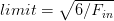$limit = sqrt{6 / F_{in}}$, where Fin is the number of input units in the layer:

```>>> F_in = 64
>>> F_out = 32
>>> limit = np.sqrt(6 / float(F_in))
>>> W = np.random.uniform(low=-limit, high=limit, size=(F_in, F_out))```

We can also use a normal distribution as well by setting µ = 0 and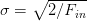$sigma = sqrt{2/ F_{in}}$

```>>> F_in = 64
>>> F_out = 32
>>> limit = np.sqrt(2 / float(F_in))
>>> W = np.random.normal(0.0, limit, size=(F_in, F_out))```

### Differences in Initialization Implementation

The actual `limit` values may vary for LeCun Uniform/Normal, Xavier Uniform/Normal, and He et al. Uniform/Normal. For example, when using Xavier Uniform in Caffe, `limit = np.sqrt(3/n)` (Heinrich, 2015), where n is either the Fin, Fout, or their average.

On the other hand, the default Xaiver initialization for Keras uses `np.sqrt(6/(F_in + F_out))` (Keras contributors, 2016). No method is “more correct” than the other, but you should read the documentation of your respective deep learning library.

### What's next? I recommend PyImageSearch University.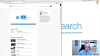Course information:
15 total classes • 23h 32m video • Last updated: 4/2021
★★★★★ 4.84 (128 Ratings) • 3,690 Students Enrolled

I strongly believe that if you had the right teacher you could master computer vision and deep learning.

Do you think learning computer vision and deep learning has to be time-consuming, overwhelming, and complicated? Or has to involve complex mathematics and equations? Or requires a degree in computer science?

That’s not the case.

All you need to master computer vision and deep learning is for someone to explain things to you in simple, intuitive terms. And that’s exactly what I do. My mission is to change education and how complex Artificial Intelligence topics are taught.

If you're serious about learning computer vision, your next stop should be PyImageSearch University, the most comprehensive computer vision, deep learning, and OpenCV course online today. Here you’ll learn how to successfully and confidently apply computer vision to your work, research, and projects. Join me in computer vision mastery.

Inside PyImageSearch University you'll find:

• 15 courses on essential computer vision, deep learning, and OpenCV topics
• � 15 Certificates of Completion
• 23h 32m on-demand video
• Brand new courses released every month, ensuring you can keep up with state-of-the-art techniques
• Pre-configured Jupyter Notebooks in Google Colab
• � Run all code examples in your web browser — works on Windows, macOS, and Linux (no dev environment configuration required!)
• � Access to centralized code repos for all 400+ tutorials on PyImageSearch
• Easy one-click downloads for code, datasets, pre-trained models, etc.
• � Access on mobile, laptop, desktop, etc.

## Summary

In this tutorial, we reviewed the fundamentals of neural networks. Specifically, we focused on the history of neural networks and the relation to biology.

From there, we moved on to artificial neural networks, such as the Perceptron algorithm. While important from a historical standpoint, the Perceptron algorithm has one major flaw — it cannot accurately classify nonlinear separable points. In order to work with more challenging datasets we need both (1) nonlinear activation functions and (2) multi-layer networks.

To train multi-layer networks we must use the backpropagation algorithm. We then implemented backpropagation by hand and demonstrated that when used to train multi-layer networks with nonlinear activation functions, we can model nonlinearly separable datasets, such as XOR.

Of course, implementing backpropagation by hand is an arduous process prone to bugs — we, therefore, often rely on existing libraries such as Keras, Theano, TensorFlow, etc. This enables us to focus on the actual architecture rather than the underlying algorithm used to train the network.

Finally, we reviewed the four key ingredients when working with any neural network, including the dataset, loss function, model/architecture, and optimization method.

Unfortunately, as some of our results demonstrated (e.g., CIFAR-10) standard neural networks fail to obtain high classification accuracy when working with challenging image datasets that exhibit variations in translation, rotation, viewpoint, etc. In order to obtain reasonable accuracy on these datasets, we’ll need to work with a special type of feedforward neural networks called Convolutional Neural Networks (CNNs), which we will cover in a separate tutorial.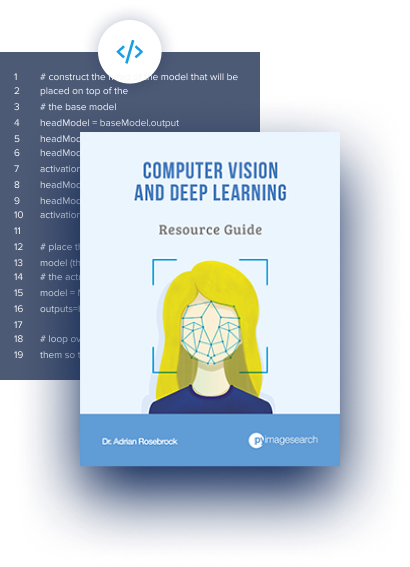#### Download the Source Code and FREE 17-page Resource Guide

Enter your email address below to get a .zip of the code and a FREE 17-page Resource Guide on Computer Vision, OpenCV, and Deep Learning. Inside you'll find my hand-picked tutorials, books, courses, and libraries to help you master CV and DL!